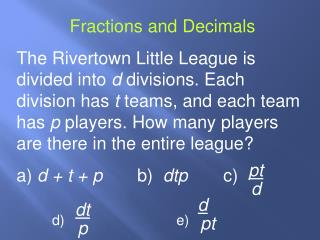DownloadDownload PresentationFractions and Decimals

Fractions and Decimals

Télécharger la présentationFractions and Decimals

- - - - - - - - - - - - - - - - - - - - - - - - - - - E N D - - - - - - - - - - - - - - - - - - - - - - - - - - -
Presentation Transcript

1. dt pt d p d pt Fractions and Decimals The Rivertown Little League is divided into d divisions. Each division has t teams, and each team has p players. How many players are there in the entire league? a) d + t + p b) dtp c) d) e)

2. Fractions and Decimals • What is the value of n if 2n+1 = 32? • 4 b) 5 c) 6 • d) 7 e) 8

3. 15# #2 Fractions and Decimals • If, for any number b, b# = b + 1 and #b = b – 1, which of the following is NOT equal to (3#)(#5)? • (1#)(#9) b) 7# + #9 • c) (4#)(#4) d) (7#)(#3) • e)

4. Fractions and Decimals • If a is a multiple of 5 and b = 5a, which of the following could be the value of a + b? i. 60 • ii. 100 • iii. 150 • i only b) iii only c) i and iii only • d) ii and iii only e) i, ii, and iii

5. Fractions and Decimals • Which of the following CANNOT be expressed as the sum of two or more consecutive positive integers? • 17 b) 22 c) 24 • d) 26 e) 32

6. 28 3 Fractions and Decimals • Which of the following is a solutin of the equations 3│x│+ 5 = 23? • -6 b) -4 c) • d) 15 e) The equation has no solution

7. Fractions and Decimals • The operation ** is defined as follows: For any positive numbers a and b, a**b= • 11**5 b) 4**9 c) 4**16 • d) 7**4 e) 9**9

8. Fractions and Decimals • What is the value of n if • 310 x 272 = 92 x 3n? • 6 b) 10 c) 12 • d) 15 e) 30

9. Fractions and Decimals If A is the sum of the integers from 1 to 50 and B is the sum of the integers from 51 to 100, what is the value of B-A?

10. Fractions and Decimals • Which of the following CANNOT be expressed as the sum of three consecutive integers? • 18 b) 24 c) 28 • d) 33 e) 36